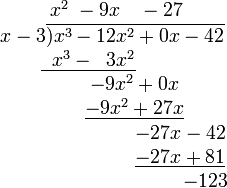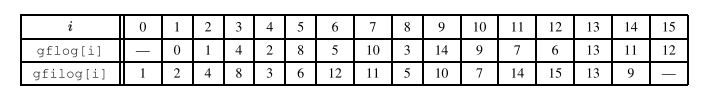# kk Blog —— 通用基础

## nginx https/nginx 配置

curl wget 不验证证书进行https请求

#### 服务端

test_private.perm 是私匙, test.crt 是证书

vim /etc/nginx/nginx.conf

curl 参数带证书

## git tag常用操作

https://blog.csdn.net/albertsh/article/details/63253614

#### 标签分类

git标签分为两种类型：轻量标签和附注标签。轻量标签是指向提交对象的引用，附注标签则是仓库中的一个独立对象，建议使用附注标签，日后还可以查看标签信息。

## 生成树计数

### SRM733-DIV1-B

https://community.topcoder.com/stat?c=round_stats&rd=17140&dn=1

#### Problem Statement

Consider an undirected, complete, simple graph G on n vertices. The vertices of G are labeled from 1 to n. Specifically, each pair of distinct vertices is connected by a single undirected edge, so there are precisely n*(n-1)/2 edges in this graph.

You are given a set E that contains some edges of the graph G. More precisely, you are given the vector s x and y. For each valid i, (x[i], y[i]) is one of the edges in E.

A spanning tree of G is a subset of exactly n-1 edges of G such that the edges connect all n vertices. You may note that the edges of a spanning tree do indeed form a tree that is a subgraph of G and spans all its vertices.

We are interested in spanning trees that contain all of the edges in the provided set E. Compute and return the number of such spanning trees modulo 987,654,323. Two spanning trees are different if there is an edge of G that is in one of them but not in the other. Definition

#### Class:

BuildingSpanningTreesDiv1

#### Method:

getNumberOfSpanningTrees

#### Parameters:

int, vector , vector

int

#### Method signature:

int getNumberOfSpanningTrees(int n, vector x, vector y)

(be sure your method is public)

#### Limits

Time limit (s): 2.000

Memory limit (MB): 256

#### Notes

987,654,323 is a prime number.

#### Constraints

n will be between 1 and 1,000, inclusive.
x will contain between 1 and 1,000 elements, inclusive.
y will contain between 1 and 1,000 elements, inclusive.
x and y will contain the same number of elements.
Each element of x will be between 1 and n-1, inclusive.
Each element of y will be between 2 and n, inclusive.
For each valid i, x[i] will be less than y[i].
All edges in E will be distinct.

#### Examples

0)
3
{1,2}
{2,3}
Returns: 1
The edges in the provided set E alredy form a spanning tree, so there is exactly one spanning tree that contains them.

1)
5
{1,3,4}
{2,4,5}
Returns: 6
There are six ways to add the one missing edge: one endpoint of the new edge must lie in the set {1,2} and the other in the set {3,4,5}.

2)
4
{1}
{2}
Returns: 8
There are eight spanning trees that contain the edge (1, 2):
{(1, 2), (1, 3), (1, 4)}
{(1, 2), (1, 3), (2, 4)}
{(1, 2), (1, 3), (3, 4)}
{(1, 2), (2, 3), (2, 4)}
{(1, 2), (1, 4), (2, 3)}
{(1, 2), (1, 4), (3, 4)}
{(1, 2), (2, 3), (3, 4)}
{(1, 2), (2, 4), (3, 4)}

3)
4
{1,2,1}
{2,3,3}
Returns: 0
The set E contains a cycle, and thus there is no spanning tree that contains all these edges.

4)
8
{1,4,2,3,5}
{4,7,6,5,8}
Returns: 144

5)
1000
{1}
{2}
Returns: 529013784

Don’t forget to take the modulo.

This problem statement is the exclusive and proprietary property of TopCoder, Inc. Any unauthorized use or reproduction of this information without the prior written consent of TopCoder, Inc. is strictly prohibited. ©2003, TopCoder, Inc. All rights reserved.

## 逆元

https://blog.csdn.net/baidu_35643793/article/details/75268911

## 伽罗华域（Galois Field）上的四则运算

https://blog.csdn.net/shelldon/article/details/54729687

Évariste Galois ，伽罗华（也译作伽瓦罗），法国数学家，群论的创立者。用群论彻底解决了根式求解代数方程的问题，而且由此发展了一整套关于群和域的理论。 本文介绍伽罗华域，以及在伽罗华域上的四则运算方式。伽罗华域上的四则运算实际上是多项式计算，后文中详细介绍。

### 一、相关数学概念

#### 3、单位元

Identity Element，也叫幺元（么元），通常使用e来表示单位元。单位元和其他元素结合时，并不会改变那些元素。 对于二元运算，若ae=a，e称为右单位元；若ea=a，e称为左单位元，若ae=e*a=a，则e称为单位元。

### 二、多项式运算

#### 3、多项式除法#### 4、GF（2w）上的多项式运算

GF（2w）的多项式减法等于加法，例如x ^4 – x4就等于x4 + x4

### 三、伽罗华域

#### 2、有限域GF(2w)：

GF(p)中p必须是一个素数，才能保证集合中的所有元素都有加法和乘法逆元(0除外)。但实际应用中，很多场合需要 0到255这256个数字能组成一个域。但256不是素数，小于256的最大素数为251，如果直接把大于等于251的数截断为250，则会丢失一部分数据。

### 四、本原多项式

GF(23)上有不只一个本原多项式，选一个本原多项式x3+x+1，这8个多项式进行四则运算后 mod (x3+x+1)的结果都是8个之中的某一个。因此这8个多项式构成一个有限域。#### 通过本原多项式生成元素

1、给定一个初始集合，包含0,1和元素x，即 {0,1,x}；
2、将这个集合中的最后一个元素，即x，乘以x，如果结果的度大于等于w，则将结果mod P(x)后加入集合；
3、直到集合有2w个元素，此时最后一个元素乘以x再mod P(x)的值等于1。

 生成元素 多项式表示 二进制表示 数值表示 推导过程 0 0 0000 0 x^0 x^0 0001 1 x^1 x^1 0010 2 x^2 x^2 0100 4 x^3 x^3 1000 8 x^4 x+1 0011 3 x^3*x = x^4 mod P(x) = x+1 x^5 x^2+x 0110 6 x^4*x = (x+1)*x = x^2+x x^6 x^3+x^2 1100 12 x^7 x^3+x+1 1011 11 x^6*x = (x^3+x^2)*x = x^4 +x^3 mod P(x) = x^3+x+1 x^8 x^2+1 0101 5 x^9 x^3+x 1010 10 x^10 x^2+x+1 0111 7 x^9*x=(x^3+x)*x = x^4+x^2 mod P(x) = x^2+x+1 x^11 x^3+x^2+x 1110 14 x^12 x^3+x^2+x+1 1111 15 x^11*x=(x^3+x^2+x)*x = x^4+x^3+x^2 mod P(x) = x^3+x^2+x+1 x^13 x^3+x^2+1 1101 13 x^12*x=(x^3+x^2+1 )*x = x^4+x^3+x mod P(x)= x^3+1 x^14 x^3+1 1001 9 x^13*x=(x^3+x^2+1)*x = x^4+x^3+x mod P(x) = x^3+1 x^15 1 0001 1 x^14*x = (x^3+1)*x = x^4+x mod P(x) = 1

### 五、伽罗华域上的运算

#### 乘法和除法：

1）a7 == 0，此时结果是一个小于指数小于8的多项式，不需要取模计算。

2）a7 == 1，则将x8替换为x4 + x3 + x2 +1，而不用进行除法取模计算，结果为：

xf(x) = (x4 + x3 + x2 +1) + a6x7 + a5x6 + a4x5 + a3x4 + a2x3 + a1x1 + a0x

### 六、查表的原理

GF(2w)是一个有限域，就是元素个数是有限的，但指数k是可以无穷的。所以必然存在循环。这个循环的周期是2w-1（g不能生成多项式 0）。所以当k大于等于2w-1时，gk =gk%(2w-1)#### 查表进行乘法和除法运算的例子

7 * 9 = gfilog[gflog + gflog] = gfilog[10 + 14] = gfilog[24 mod 15] = gfilog = 10

13 / 11 = gfilog[gflog - gflog] = gfilog[13 - 7] = gfilog = 12